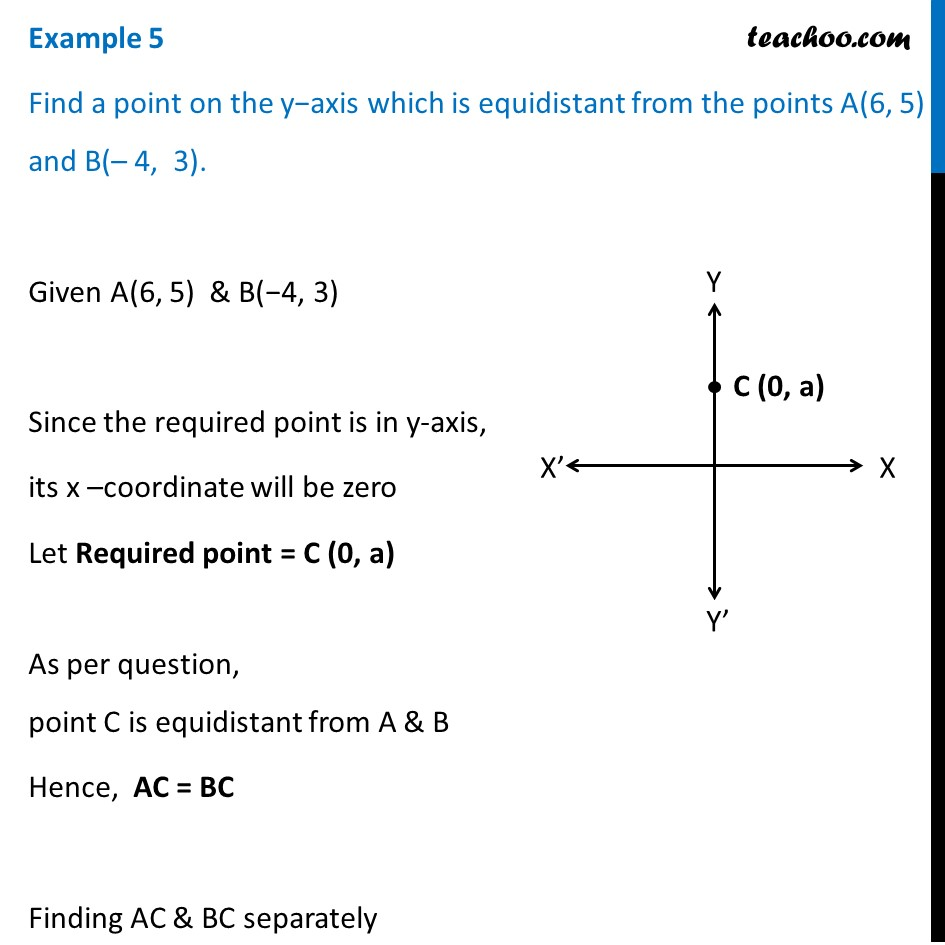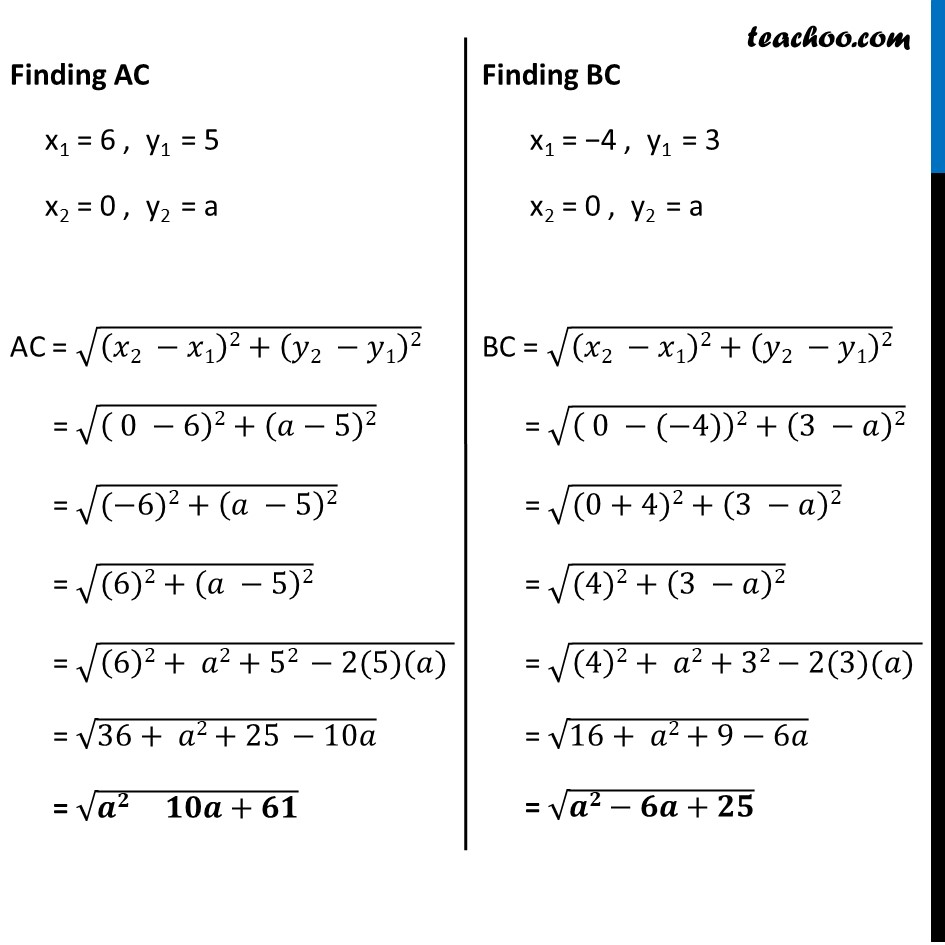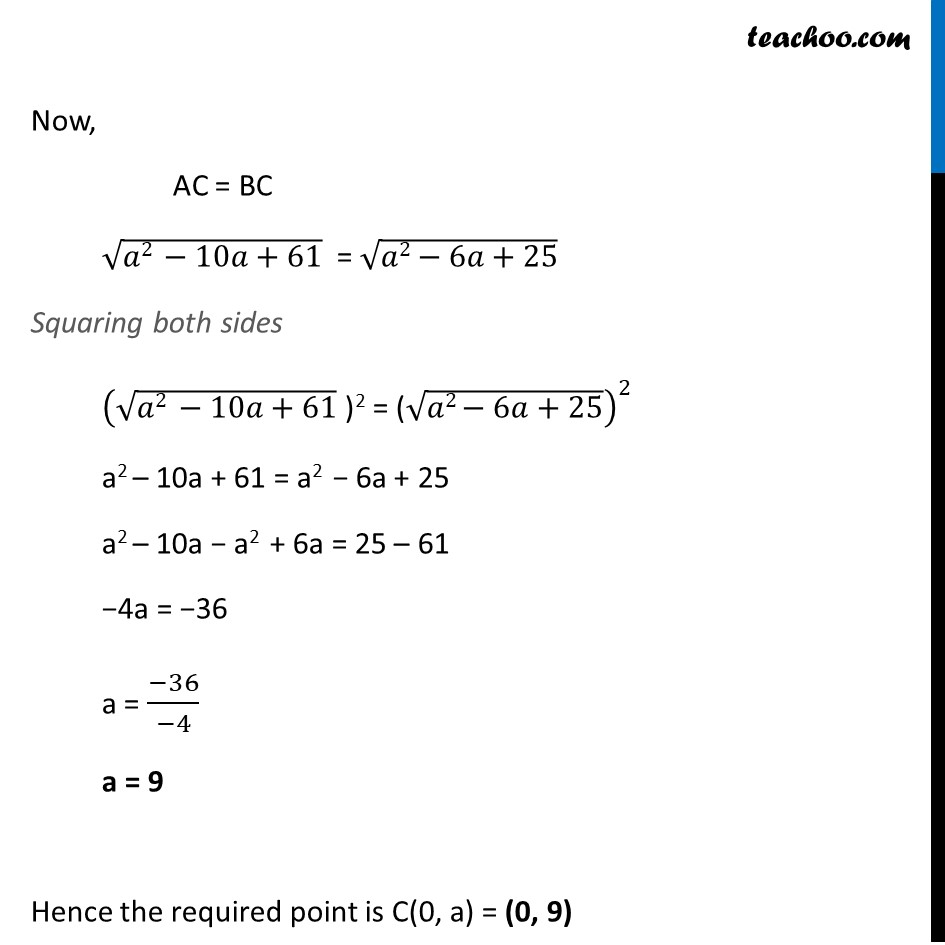Chapter 7 Class 10 Coordinate Geometry

Class 10
Important Questions for Exam - Class 10Introducing your new favourite teacher - Teachoo Black, at only ₹83 per month

### Transcript

Example 5 Find a point on the y−axis which is equidistant from the points A(6, 5) and B(– 4, 3). Given A(6, 5) & B(−4, 3) Since the required point is in y-axis, its x –coordinate will be zero Let Required point = C (0, a) As per question, point C is equidistant from A & B Hence, AC = BC Finding AC & BC separately Finding AC x1 = 6 , y1 = 5 x2 = 0 , y2 = a AC = √((𝑥2 −𝑥1)2+(𝑦2 −𝑦1)2) = √(( 0 −6)2+(𝑎−5)2) = √((−6)2+(𝑎 −5)2) = √((6)2+(𝑎 −5)2) = √((6)2+ 𝑎2+52 −2(5)(𝑎) ) = √(36+ 𝑎2+25 −10𝑎) = √(𝒂𝟐 −𝟏𝟎𝒂+𝟔𝟏) Finding BC x1 = −4 , y1 = 3 x2 = 0 , y2 = a BC = √((𝑥2 −𝑥1)2+(𝑦2 −𝑦1)2) = √(( 0 −(−4))2+(3 −𝑎)2) = √((0+4)2+(3 −𝑎)2) = √((4)2+(3 −𝑎)2) = √((4)2+ 𝑎2+32−2(3)(𝑎) ) = √(16+ 𝑎2+9−6𝑎) = √(𝒂𝟐−𝟔𝒂+𝟐𝟓) Now, AC = BC √(𝑎2 −10𝑎+61) = √(𝑎2−6𝑎+25) Squaring both sides (√(𝑎2 −10𝑎+61) " )2 = (" √(𝑎2−6𝑎+25))^2 a2 – 10a + 61 = a2 − 6a + 25 a2 – 10a − a2 + 6a = 25 – 61 −4a = −36 a = (−36)/(−4) a = 9 Hence the required point is C(0, a) = (0, 9)